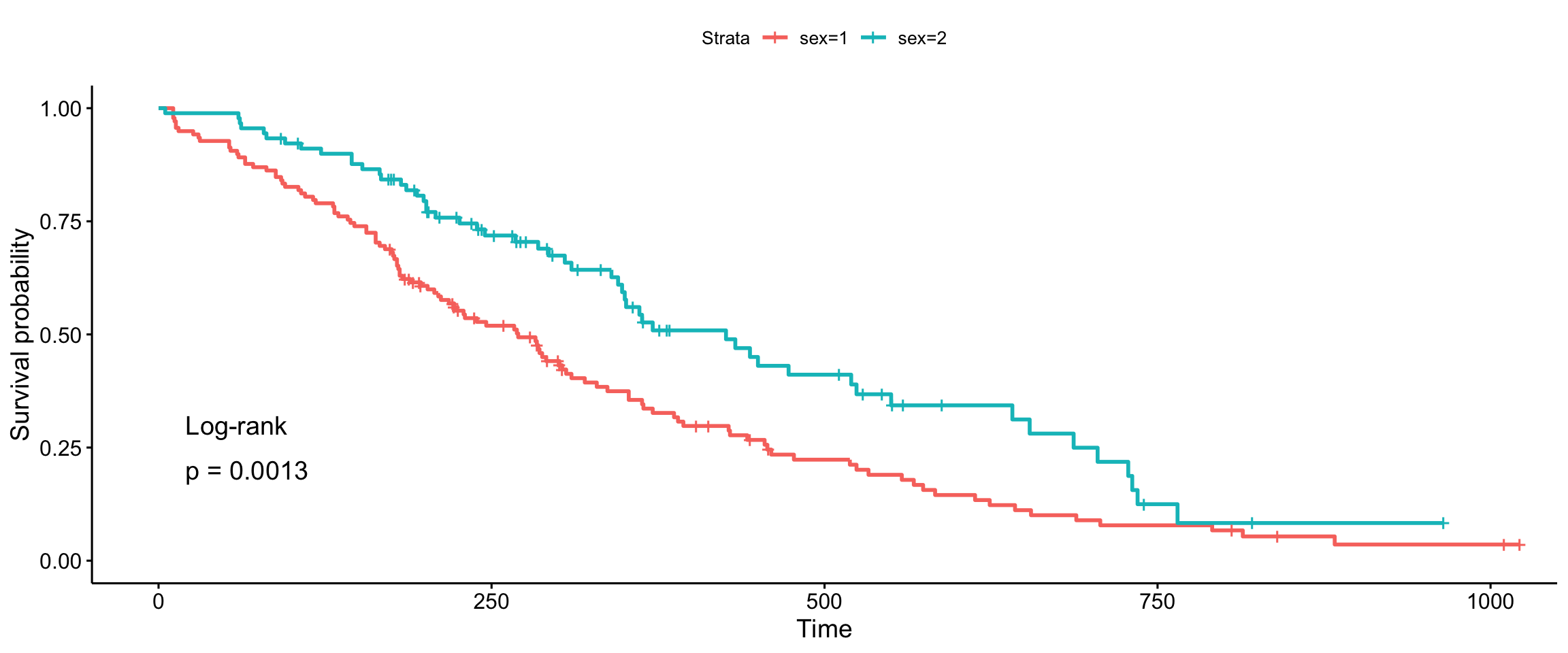library("survminer")

This vignette covers changes between versions 0.2.4 and 0.2.5 for specifiyng weights in the log-rank comparisons done in ggsurvplot().

# Log-rank statistic for 2 groups

As it is stated in the literature, the Log-rank test for comparing survival (estimates of survival curves) in 2 groups ($$A$$ and $$B$$) is based on the below statistic

$LR = \frac{U^2}{V} \sim \chi(1),$

where $U = \sum_{i=1}^{T}w_{t_i}(o_{t_i}^A-e_{t_i}^A), \ \ \ \ \ \ \ \ V = Var(U) = \sum_{i=1}^{T}(w_{t_i}^2\frac{n_{t_i}^An_{t_i}^Bo_{t_i}(n_{t_i}-o_{t_i})}{n_{t_i}^2(n_{t_i}-1)})$ and

• $$t_i$$ for $$i=1, \dots, T$$ are possible event times,
• $$n_{t_i}$$ is the overall risk set size on the time $$t_i$$ ($$n_{t_i} = n_{t_i}^A+n_{t_i}^B$$),
• $$n_{t_i}^A$$ is the risk set size on the time $$t_i$$ in group $$A$$,
• $$n_{t_i}^B$$ is the risk set size on the time $$t_i$$ in group $$B$$,
• $$o_{t_i}$$ overall observed events in the time $$t_i$$ ($$o_{t_i} = o_{t_i}^A+o_{t_i}^B$$),
• $$o_{t_i}^A$$ observed events in the time $$t_i$$ in group $$A$$,
• $$o_{t_i}^B$$ observed events in the time $$t_i$$ in group $$B$$,
• $$e_{t_i}$$ number of overall expected events in the time $$t_i$$ ($$e_{t_i} = e_{t_i}^A+e_{t_i}^B$$),
• $$e_{t_i}^A$$ number of expected events in the time $$t_i$$ in group $$A$$,
• $$e_{t_i}^B$$ number of expected events in the time $$t_i$$ in group $$B$$,
• $$w_{t_i}$$ is a weight for the statistic,

$e_{t_i}^A = n_{t_i}^A \frac{o_{t_i}}{n_{t_i}}, \ \ \ \ \ \ \ \ \ \ e_{t_i}^B = n_{t_i}^B \frac{o_{t_i}}{n_{t_i}},$ $e_{t_i}^A + e_{t_i}^B = o_{t_i}^A + o_{t_i}^B$

that’s why we can substitute group $$A$$ with $$B$$ in $$U$$ and receive same results.

# Weighted Log-rank extensions

Regular Log-rank comparison uses $$w_{t_i} = 1$$ but many modifications to that approach have been proposed. The most popular modifications, called weighted Log-rank tests, are available in ?survMisc::comp

• n Gehan and Breslow proposed to use $$w_{t_i} = n_{t_i}$$ (this is also called generalized Wilcoxon),
• srqtN Tharone and Ware proposed to use $$w_{t_i} = \sqrt{n_{t_i}}$$,
• S1 Peto-Peto’s modified survival estimate $$w_{t_i} = S1({t_i}) = \prod_{i=1}^{T}(\frac{1-e_{t_i}}{n_{t_i}+1})$$,
• S2 modified Peto-Peto (by Andersen) $$w_{t_i} = S2({t_i}) = \frac{S1({t_i})n_{t_i}}{n_{t_i}+1}$$,
• FH Fleming-Harrington $$w_{t_i} = S(t_i)^p(1 - S(t_i))^q$$.

Watch out for FH as I submitted an info on survMisc repository where I think their mathematical notation is misleading for Fleming-Harrington.

## Why are they useful?

The regular Log-rank test is sensitive to detect differences in late survival times, where Gehan-Breslow and Tharone-Ware propositions might be used if one is interested in early differences in survival times. Peto-Peto modifications are also useful in early differences and are more robust (than Tharone-Whare or Gehan-Breslow) for situations where many observations are censored. The most flexible is Fleming-Harrington method for weights, where high p indicates detecting early differences and high q indicates detecting differences in late survival times. But there is always an issue on how to detect p and q.

Remember that test selection should be performed at the research design level! Not after looking in the dataset.

# Plots

library("survival")
data("lung")
fit <- survfit(Surv(time, status) ~ sex, data = lung)

After preparing a functionality for this GitHub’s issue Other tests than log-rank for testing survival curves and Log-rank test for trend we are now able to compute p-values for various Log-rank test in survminer package. Let as see below examples on executing all possible tests.

## Log-rank (survdiff)

ggsurvplot(fit, data = lung, pval = TRUE, pval.method = TRUE)## Log-rank (comp)

ggsurvplot(fit, data = lung, pval = TRUE, pval.method = TRUE,
log.rank.weights = "1")## Gehan-Breslow (generalized Wilcoxon)

ggsurvplot(fit, data = lung, pval = TRUE, pval.method = TRUE,
log.rank.weights = "n", pval.method.coord = c(5, 0.1),
pval.method.size = 3)